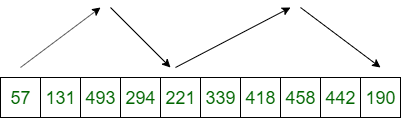Related Articles
Sort an Increasing-Decreasing Array
• Difficulty Level : Easy
• Last Updated : 28 Nov, 2019

Given a K-increasing-decreasing array arr[], the task is to sort the given array. An array is said to be K-increasing-decreasing if elements repeatedly increase upto a certain index after which they decrease, then again increase, a total of K times. The diagram below shows a 4-increasing-decreasing array.Example:

Input: arr[] = {57, 131, 493, 294, 221, 339, 418, 458, 442, 190}
Output: 57 131 190 221 294 339 418 442 458 493

Input: arr[] = {1, 2, 3, 4, 3, 2, 1}
Output: 1 1 2 2 3 3 4

## Recommended: Please try your approach on {IDE} first, before moving on to the solution.

Approach: The brute-force approach is to sort the array without taking advantage of the k-increasing-decreasing property. The time complexity of this approach is O(n logn) where n is the length of the array.

If k is significantly smaller than n, a better approach can be found with less time complexity. For example, if k=2, the input array consists of two subarrays, one increasing, the other decreasing. Reversing the second subarray yields two sorted arrays and the result is then merged which can be done in O(n) time. Generalizing, we could first reverse the order of each of the decreasing subarrays. For example, in the above figure, the array could be decomposed into four sorted arrays as {57, 131, 493}, {221, 294}, {339, 418, 458} and {190, 442}. Now the min-heap technique can be used to merge these sorted arrays.

Below is the implementation of the above approach:

 `// C++ implementation of the approach``#include ``using` `namespace` `std;`` ` `// A pair of pairs, first element is going to``// store value, second element index of array``// and third element index in the array``typedef` `pair<``int``, pair<``int``, ``int``> > ppi;`` ` `// This function takes an array of arrays as an``// argument and all arrays are assumed to be``// sorted``// It merges them together and returns the``// final sorted output``vector<``int``> mergeKArrays(vector > arr)``{``    ``vector<``int``> output;`` ` `    ``// Create a min heap with k heap nodes``    ``// Every heap node has first element of an array``    ``priority_queue, greater > pq;`` ` `    ``for` `(``int` `i = 0; i < arr.size(); i++)``        ``pq.push({ arr[i], { i, 0 } });`` ` `    ``// Now one by one get the minimum element``    ``// from min heap and replace it with next``    ``// element of its array``    ``while` `(pq.empty() == ``false``) {``        ``ppi curr = pq.top();``        ``pq.pop();`` ` `        ``// i ==> Array Number``        ``// j ==> Index in the array number``        ``int` `i = curr.second.first;``        ``int` `j = curr.second.second;`` ` `        ``output.push_back(curr.first);`` ` `        ``// The next element belongs to same array as``        ``// current``        ``if` `(j + 1 < arr[i].size())``            ``pq.push({ arr[i][j + 1], { i, j + 1 } });``    ``}`` ` `    ``return` `output;``}`` ` `// Function to sort the alternating``// increasing-decreasing array``vector<``int``> SortKIncDec(``const` `vector<``int``>& A)``{``    ``// Decompose the array into a``    ``// set of sorted arrays``    ``vector > sorted_subarrays;``    ``typedef` `enum` `{ INCREASING,``                   ``DECREASING } SubarrayType;``    ``SubarrayType subarray_type = INCREASING;``    ``int` `start_idx = 0;``    ``for` `(``int` `i = 0; i <= A.size(); i++) {`` ` `        ``// If the current subarrays ends here``        ``if` `(i == A.size()``            ``|| (A[i - 1] < A[i]``                ``&& subarray_type == DECREASING)``            ``|| (A[i - 1] >= A[i]``                ``&& subarray_type == INCREASING)) {`` ` `            ``// If the subarray is increasing``            ``// then add from the start``            ``if` `(subarray_type == INCREASING) {``                ``sorted_subarrays.emplace_back(A.cbegin() + start_idx,``                                              ``A.cbegin() + i);``            ``}`` ` `            ``// If the subarray is decreasing``            ``// then add from the end``            ``else` `{``                ``sorted_subarrays.emplace_back(A.crbegin()``                                                  ``+ A.size() - i,``                                              ``A.crbegin()``                                                  ``+ A.size()``                                                  ``- start_idx);``            ``}``            ``start_idx = i;``            ``subarray_type = (subarray_type == INCREASING``                                 ``? DECREASING``                                 ``: INCREASING);``        ``}``    ``}`` ` `    ``// Merge the k sorted arrays```    ``return` `mergeKArrays(sorted_subarrays);``}`` ` `// Driver code``int` `main()``{``    ``vector<``int``> arr = { 57, 131, 493, 294, 221,``                        ``339, 418, 458, 442, 190 };`` ` `    ``// Get the sorted array``    ``vector<``int``> ans = SortKIncDec(arr);`` ` `    ``// Print the sorted array``    ``for` `(``int` `i = 0; i < ans.size(); i++)``        ``cout << ans[i] << ``" "``;`` ` `    ``return` `0;``}`
Output:
```57 131 190 221 294 339 418 442 458 493
```

Time Complexity: O(n*logk), where n is the length of array.My Personal Notes arrow_drop_up